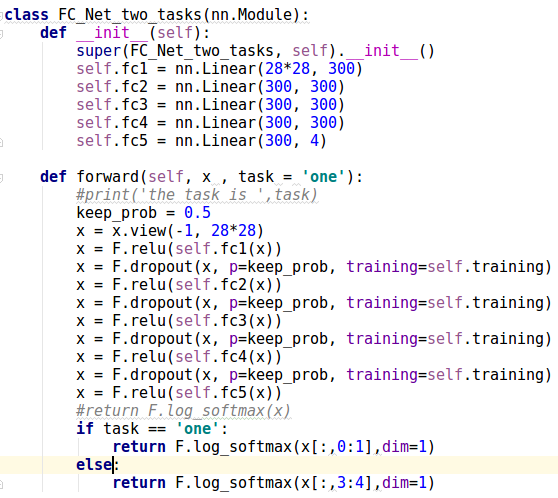# Multitask Sequential learningI want to return the log_softmax function according to the task indicator like this
if task == ‘one’:
return F.log_softmax(x[:,0:1],dim=1)
else:
return F.log_softmax(x[:,3:4],dim=1)

But I got following errors:
Traceback (most recent call last):
File “adversarial_FC_Network.py”, line 393, in
train(epoch)
File “adversarial_FC_Network.py”, line 363, in train
loss.backward()
File “/home/shixian/.local/lib/python3.5/site-packages/torch/autograd/variable.py”, line 167, in backward
File “/home/shixian/.local/lib/python3.5/site-packages/torch/autograd/init.py”, line 99, in backward
RuntimeError: cublas runtime error : an internal operation failed at /pytorch/torch/lib/THC/THCBlas.cu:246

Here is the code of my module:
def init(self):
self.fc1 = nn.Linear(28*28, 300)
self.fc2 = nn.Linear(300, 300)
self.fc3 = nn.Linear(300, 300)
self.fc4 = nn.Linear(300, 300)
self.fc5 = nn.Linear(300, 4)

``````def forward(self, x , task = 'one'):
keep_prob = 0.5
x = x.view(-1, 28*28)
x = F.relu(self.fc1(x))
x = F.dropout(x, p=keep_prob, training=self.training)
x = F.relu(self.fc2(x))
x = F.dropout(x, p=keep_prob, training=self.training)
x = F.relu(self.fc3(x))
x = F.dropout(x, p=keep_prob, training=self.training)
x = F.relu(self.fc4(x))
x = F.dropout(x, p=keep_prob, training=self.training)
x = F.relu(self.fc5(x))
#return F.log_softmax(x)
if task == 'one':
return F.log_softmax(x[:,0:1],dim=1)
else:
return F.log_softmax(x[:,3:4],dim=1)``````

I made a simple mistakes.
It should be return F.log_softmax(x[:,0:2],dim=1)
I do not know how to close this post sorry